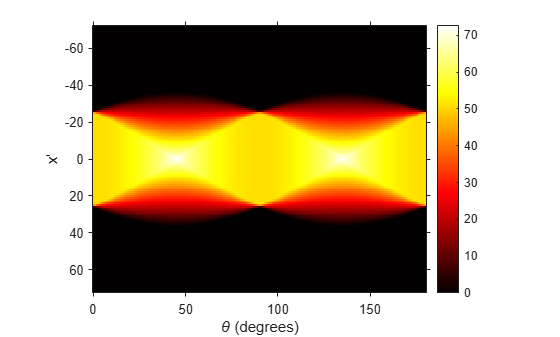Main Content

# radon

Radon transform

## Syntax

R = radon(I)
R = radon(I,theta)
[R,xp] = radon(___)

## Description

R = radon(I) returns the Radon transform R of 2-D grayscale image I for angles in the range [0, 179] degrees. The Radon transform is the projection of the image intensity along a radial line oriented at a specific angle.

example

R = radon(I,theta) returns the Radon transform for the angles specified by theta.
[R,xp] = radon(___) returns a vector xp containing the radial coordinates corresponding to each row of the image.

## Examples

collapse all

Make the axes scale visible for this image.

iptsetpref('ImshowAxesVisible','on')

Create a sample image.

I = zeros(100,100); I(25:75, 25:75) = 1;

Calculate the Radon transform.

theta = 0:180; [R,xp] = radon(I,theta);

Display the transform.

imshow(R,[],'Xdata',theta,'Ydata',xp,'InitialMagnification','fit') xlabel('\theta (degrees)') ylabel('x''') colormap(gca,hot), colorbarMake the axes scale invisible.

iptsetpref('ImshowAxesVisible','off')

## Input Arguments

collapse all

Grayscale image, specified as a 2-D numeric matrix.

Data Types: single | double | int8 | int16 | int32 | int64 | uint8 | uint16 | uint32 | uint64 | logical

Projection angles in degrees, specified as a numeric scalar or numeric vector.

Data Types: double

## Output Arguments

collapse all

Radon transform of image I, returned as one of the following.

• If theta is a scalar, then R is a numeric column vector containing the Radon transform for theta degrees.

• If theta is a vector, then R is a matrix in which each column is the Radon transform for one of the angles in theta.

Radial coordinates corresponding to each row of R, returned as a numeric vector. The radial coordinates are the values along the x'-axis, which is oriented at theta degrees counterclockwise from the x-axis. The origin of both axes is the center pixel of the image, which is defined as

floor((size(I)+1)/2)
For example, in a 20-by-30 image, the center pixel is (10,15).

## Algorithms

The Radon transform of an image is the sum of the Radon transforms of each individual pixel.

The algorithm first divides pixels in the image into four subpixels and projects each subpixel separately, as shown in the following figure.Each subpixel's contribution is proportionally split into the two nearest bins, according to the distance between the projected location and the bin centers. If the subpixel projection hits the center point of a bin, the bin on the axes gets the full value of the subpixel, or one-fourth the value of the pixel. If the subpixel projection hits the border between two bins, the subpixel value is split evenly between the bins.

 Bracewell, Ronald N., Two-Dimensional Imaging, Englewood Cliffs, NJ, Prentice Hall, 1995, pp. 505-537.

 Lim, Jae S., Two-Dimensional Signal and Image Processing, Englewood Cliffs, NJ, Prentice Hall, 1990, pp. 42-45.

Download ebook